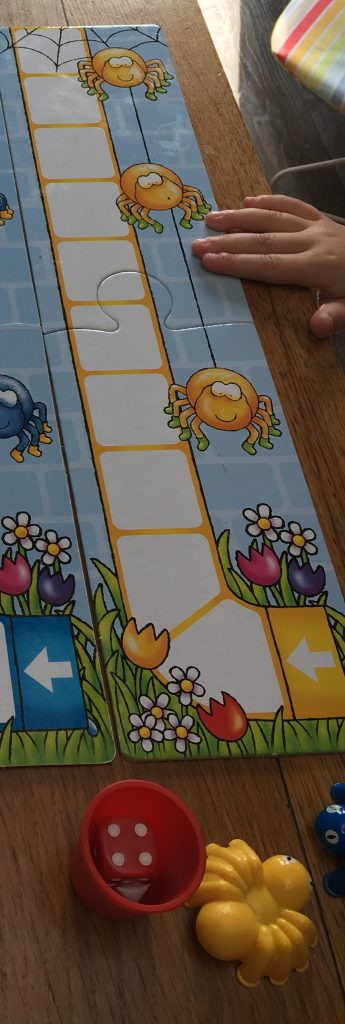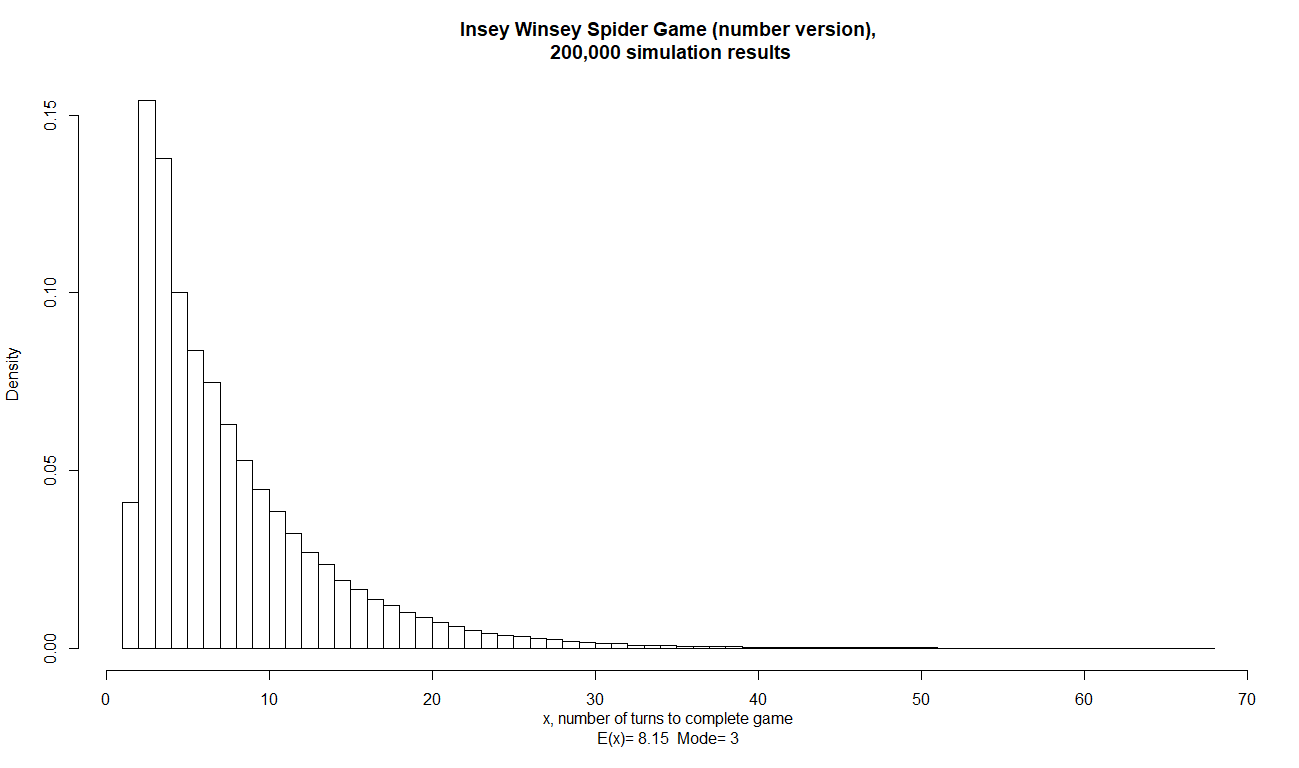# Insey Winsey Spider Game Monte-Carlo Simulation

Time is such a precious commodity especially with a family. So when your daughter asks to play a board game… you think ‘how long will this take’. With most board games, one is able to roughly estimate how long the game will take… the Shopping Game, well that can be expected to take 10 minutes with 2 players. The Memory Game, well that can be expected to take 15 minutes with 2 players. Whilst there is of course some variation, I have found most (age 4-6) games to have a fairly symmetrical and tight distribution of time taken to complete the game.

One exception though, has been the Insey Winsey Spider Game… where saying yes to a game has derailed many’s a schedule or upset a child because it can very frequently take much longer than expected… one time it’s all over in 3 moves, next time it takes 20 moves. So, let’s try out some Monte-Carlo simulation modelling in R to understand the distribution of moves better!As a player in the game, you are provided with a waterspout and a spider. The spider starts at the bottom of the drain spout. Each turn:

you roll the six-sided die and progress your spider upwards by the number of squares awarded by the die. you spin a spinner to determine the weather: sunshine or rain. Rain takes up approx 30% of the spinner’s space and when it does happen, your spider gets washed out back to the bottom of the waterspout. The winner is the first to exit the top of the spout. We’re interested in how many turns this can take!

In terms of doing some Monte-Carlo simulation modelling in R, our strategy will be to model a turn (the roll of the six-sided die and the spin of the spinner); then model an entire game – keeping on going until our spider has reached more than 10 squares from the start; and record the number of moves taken – as that’s what we’re curious about!

``````#RETURNS RESULT OF ROLL OF A 6 SIDED DIE
six_sided_number_die_roll <- function()
return(sample(x=c(1:6),size = 1,replace = TRUE))
``````

Using the sample function in R, we are able to take a random sample of the sequence 1:6 with replacement.

``````#RETURNS RESULT OF SPIN OF SPECIAL WEATHER SPINNER
weather_spin <- function()
return(sample(x=c('raining','sunshine'),size=1,replace = TRUE, prob = c(0.3,0.7)))
``````

For the spinner, we wish to table a random sample of ‘raining’ and ‘sunshine’, again with replacement, but this time specifying uneven odds of 30% and 70% respectively through the prob argument.

Now that we have created two functions that enable us to model a turn, let’s bring these together in a full game simulation.

``````#SIMULATES ONE FULL GAME OF 'INSEY WINSEY SPIDER', NUMBER VERSION
spider_game_number_run <- function() {
spider_position = 0
i = 0
while (spider_position <= 10)
{
spider_position <- spider_position +six_sided_number_die_roll()
if(weather_spin() == 'raining') spider_position <- 0
i = i + 1
}
return(i)
}
``````

We set up two counters: spider_position which is going to count how many squares up the waterspout the spider is; and i which is the number of turns taken. With both of these counters set to zero, we are then ready to start our while loop which will continue while the spider’s position is less than or equal to 10 squares. During that time, the spider’s position is incremented by one die roll, and one weather spin is made. If the weather spin value is raining then the spider’s position is reset to 0. Once this while loop completes, the value returned is i, the number of turns taken to reach completion for that simulated game.

Now we can simulate an entire game by calling the function spider_game_number_run() and it will return the number of turns taken to complete. What we need to do now is run this simulation many times to understand the distribution of turns needed to complete a game.

``````#SIMULATES k FULL GAMES OF 'INSEY WINSEY SPIDER'
spider_game_number_sim <- function(k) {
j = 1
agg <- NULL
while(j < k + 1)
{agg[j] <- spider_game_number_run()
j = j + 1}
#return the number of turns it took to finish
return(agg)
}
``````

Our function takes the argument k and will run the game simulation k-times, each time adding the number of turns taken to the agg object which it returns. With this in hand, finally we want to simulate the game a good number of times and display the results.

``````n=200000
start_time <- Sys.time()
a <- spider_game_number_sim(n)
elapsed_time <- Sys.time() - start_time
a_mode <- getmode(a)
a_mean <- mean(a)
hist(a, freq = FALSE, breaks = seq(1:max(a)),
main = paste("Insey Winsey Spider Game (number version),\n", format(n,scientific = FALSE, big.mark = ","),"simulation results"),
xlab = paste("x, number of turns to complete game\nE(x)=",round(a_mean,2)," Mode=",a_mode))
``````

All of this code (plus a little bit more) can be found on the Github repository for this blog, https://github.com/statsonthecloud/blog. The code above completed in around 50s on a modest low-powered Intel laptop. The output was the following histogram.Distribution of number of turns.

So time for a sense check: the waterspout is 10 squares tall, and if one is particularly lucky, the spider can progress 10 in two goes (6+6,5+6,6+5, and two sunshine spins). The minimum is two goes to complete the game – this is accurately represented on our graph.

The mode number of turns is 3. OK, but perhaps this is not so helpful given the large spread of possible turns. More meaningful perhaps is the expected number of turns, which is 8.15. 50% of the time, we expect games to take up to 8 turns; 50% of the time we expect it will take longer than 8 turns. But what fate are we likely to face (in terms of parental time management) if we end up taking more than 8 turns. Well, the distribution has a long tail, so it could well end up taking many more turns. That long tail was what I felt was happening, and through a quick’n’dirty Monte-Carlo simulation in R, I was able to thoroughly explore and visualise this behaviour!

The beauty of the simulation approach is that you can arrive at answers quickly. However it would also probably be possible to do this mathematically and to propose a distribution analytically – maybe that can be a part II to this post one day in the future. For now, I think I have to go as I’m being called to play a game…